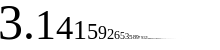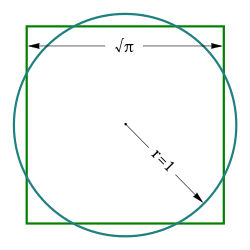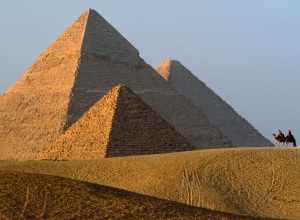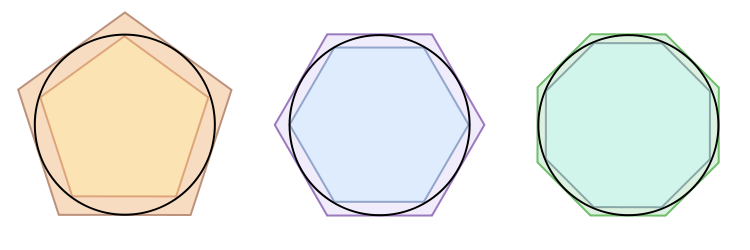Circles I: π pie

“Well, I had a vision when I was twelve. And I saw a man on a flaming pie, and he said, ‘You are the Beatles with an A.’ And so we are.”
CBS News: Out of a flaming pie comes The Beatles

“We can conclude that although the ancient Egyptians could not precisely define the value of π, in practice they used it”.
– Verner, M. (2003). The Pyramids: Their Archaeology and History, p. 70.

The Sun as flaming π: irrational & transcendentalIn symbolic code, the Sun is represented by a dot, a hole, a disc, a ring, 360°. The Sun is Ra’s flaming Eye. The Sun is also represented by various types of food, including pizza and pie. “Pie” has multiple meanings, and one of those meanings is a play on π (Pi), the mathematical representation of the ratio of a circle’s circumference to its diameter.π is an irrational number and cannot be expressed by a common fraction, but a close approximation would be 22/7. π is expressed as a decimal which neither ends nor repeats itself. It is a transcendental number (Wikipedia).Because π is a transcendental number, it is impossible to construct, using compass and straightedge, a square whose area is equal to the area of a given circle. Squaring a circle, one of the important geometry problems of the classical antiquity, was finally proven impossible in 1882 by Ferdinand von Lindemann. Over the years, the concept of “squaring the circle” has been used by writers like Dante and Pope as a metaphor for attempting an impossible task.

History of πThe earliest written approximations of π are found in Egypt (Rhind Papyrus, 1650 BC-1850 B.C.) and Babylon (a clay tablet dated 1900–1600 B.C.) both within 1 percent of the true value (Wikipedia: π). Based on ratios in the Great Pyramid at Giza (~2550 B.C.), some Egyptologists have concluded that the pyramid builders had knowledge of π and deliberately designed the pyramid to incorporate the proportions of a circle (Ibid).

Inscribing and circumscribing a circle with a 96-sided polygon, Archimedes (Sicily ~287-212 B.C.) gave an approximation of the value of pi (π), showing it to be greater than 223⁄71 and less than 22⁄7 (Archimedes On the Measurement of a Circle)Thoughts on π, squaring the circle, and TarotBased on number relationships in the angles of various types of polygons which I wrote about in Number 9 Geometry, I believe the Tarot deck is based at least partly on geometric concepts. With that in mind, it is interesting that π’s closest fraction is 22/7, since one of the aspects of the Sun is The Fool (22).Maybe there’s also some subtext of frustration here relating to the frustrating, and ultimately impossible, problem of “squaring the circle.”

Perhaps related, Joseph Heller used the term “Catch-22” to represent an inescapable logical trap in his 1961 novel of the same name. The concept of an inescapable paradox connects the Catch-22 with The Fool and the notion of an impossible task (squaring the circle).

“Catch-22 says they have a right to do anything we can’t stop them from doing.”

Angle Degree Relationships and The Sun“The original motivation for choosing the degree as a unit of rotations and angles is unknown. One theory states that it is related to the fact that 360 is approximately the number of days in a year. Ancient astronomers noticed that the sun, which follows through the ecliptic path over the course of the year, seems to advance in its path by approximately one degree each day. Some ancient calendars, such as the Persian calendar, used 360 days for a year. The use of a calendar with 360 days may be related to the use of sexagesimal (base 60) numbers…

Another theory is that the Babylonians subdivided the circle using the angle of an equilateral triangle as the basic unit and further subdivided the latter into 60 parts following their sexagesimal numeric system. The earliest trigonometry, used by the Babylonian astronomers and their Greek successors, was based on chords of a circle. A chord of length equal to the radius made a natural base quantity. One sixtieth of this, using their standard sexagesimal divisions, was a degree…

The division of the circle into 360 parts also occurred in ancient India, as evidenced in the Rigveda.”

– from Wikipedia Degree (angle)A circle with an equilateral chord (red). One sixtieth of this arc is a degree. Six such chords complete the circle.

equilateral triangle 3 corners x 60° = 180°
square 4 corners x 90° = 360°
circle 0 corners = 360°